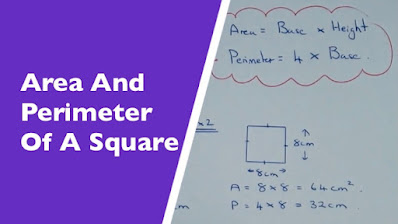# How to calculate perimeter and area of square in Java? Example Tutorial

Aprodesoft
If you are looking for a solution of problem how to calculate perimeter and area of a given Square in Java then you have come at the right place. In this article, I have given step by step solution of this common coding problem. This was actually a homework exercise when I was learning Java program and since I was good at Maths, I know how to calculate Perimeter and Area of Circle and Square but big challenge for me was to convert that knowledge into code. Another big challenge for me was how to take input from user actually that was the mistake I made when I first solved this problem.

I have to simply write a method which accept side of Square and return area and perimeter, without worrying about user input, once you have that method ready, you can think of user input part but I made that mistake and spent a lot of time figuring out how to read data from console in Java. If you are started with coding, learn from that only focus on logic first.## Java Program to calculate perimeter and area of square in Java

Anyway, here is complete Java Program to calculate area and perimeter of a Square in Java:

`import java.util.Scanner;/** Java Program to calculate the Perimeter and Area of a Square*/public class Main {public static void main(String args[]) {// creating scanner to accept radius of circleScanner scanner = new Scanner(System.in);System.out.println("Welcome in Java program to calculate the Perimeter and area of square");System.out.println("Please enter length or side of square :");int side = scanner.nextInt();int perimeter = perimeter(side);int area = area(side);System.out.println("perimeter of square: " + perimeter);System.out.println("area of square: " + area);scanner.close();}/*** Java method to calculate perimeter of a square* * @param side* @return perimeter of square*/public static int perimeter(int side) {return 4 * side;}/*** Java method to calculate area of a square* * @param side* @return area of square*/public static int area(int side) {return side * side;}}OutputWelcome in Java program to calculate the Perimeter and area of squarePlease enter length or side of square :2perimeter of square: 8area of square: 4`

You can see that when use entered side of square as two, our program correctly printed that area would be 4 which side * side and perimeter would be 8 which is four times of side.

That’s all about how to calculate Area and Perimeter of a given Square in Java. If you are learning coding then you should solve these kind of problem to first learn how to convert your thoughts and knowledge to code. Rest can follow

Some more Java Coding Exercise for Programmer

\

Aprodesoft
saya seorang yang bisa diadalakan dalam mencari rekomendasi atau informasi yang bagus untuk anda.